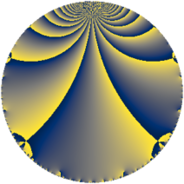# Properties

 Label 63.3.rLevel $63$ Weight $3$ Character orbit 63.r Rep. character $\chi_{63}(29,\cdot)$ Character field $\Q(\zeta_{6})$ Dimension $24$ Newform subspaces $1$ Sturm bound $24$ Trace bound $0$

# Related objects

## Defining parameters

 Level: $$N$$ $$=$$ $$63 = 3^{2} \cdot 7$$ Weight: $$k$$ $$=$$ $$3$$ Character orbit: $$[\chi]$$ $$=$$ 63.r (of order $$6$$ and degree $$2$$) Character conductor: $$\operatorname{cond}(\chi)$$ $$=$$ $$9$$ Character field: $$\Q(\zeta_{6})$$ Newform subspaces: $$1$$ Sturm bound: $$24$$ Trace bound: $$0$$

## Dimensions

The following table gives the dimensions of various subspaces of $$M_{3}(63, [\chi])$$.

Total New Old
Modular forms 36 24 12
Cusp forms 28 24 4
Eisenstein series 8 0 8

## Trace form

 $$24 q + 2 q^{3} + 24 q^{4} - 18 q^{5} - 14 q^{6} + 26 q^{9} + O(q^{10})$$ $$24 q + 2 q^{3} + 24 q^{4} - 18 q^{5} - 14 q^{6} + 26 q^{9} - 18 q^{11} + 4 q^{12} - 10 q^{15} - 48 q^{16} - 62 q^{18} - 24 q^{19} - 18 q^{20} - 14 q^{21} - 24 q^{22} + 72 q^{23} + 54 q^{24} + 54 q^{25} - 124 q^{27} + 54 q^{29} - 212 q^{30} + 30 q^{31} + 126 q^{32} - 178 q^{33} + 60 q^{34} + 124 q^{36} + 84 q^{37} - 144 q^{38} + 92 q^{39} - 60 q^{40} + 180 q^{41} + 140 q^{42} - 60 q^{43} - 118 q^{45} - 168 q^{46} + 378 q^{47} + 436 q^{48} - 84 q^{49} - 378 q^{50} + 168 q^{51} - 18 q^{52} + 514 q^{54} - 132 q^{55} - 232 q^{57} + 90 q^{58} - 90 q^{59} + 76 q^{60} + 28 q^{63} + 324 q^{64} + 126 q^{65} + 202 q^{66} + 6 q^{67} - 738 q^{68} - 432 q^{69} - 246 q^{72} - 72 q^{73} - 792 q^{74} + 40 q^{75} + 84 q^{76} + 28 q^{78} - 6 q^{79} - 34 q^{81} - 108 q^{82} - 558 q^{83} - 322 q^{84} + 126 q^{85} + 90 q^{86} + 428 q^{87} + 168 q^{88} - 488 q^{90} + 84 q^{91} + 774 q^{92} - 738 q^{93} - 354 q^{94} + 648 q^{95} - 280 q^{96} - 270 q^{97} + 296 q^{99} + O(q^{100})$$

## Decomposition of $$S_{3}^{\mathrm{new}}(63, [\chi])$$ into newform subspaces

Label Dim $A$ Field CM Traces $q$-expansion
$a_{2}$ $a_{3}$ $a_{5}$ $a_{7}$
63.3.r.a $24$ $1.717$ None $$0$$ $$2$$ $$-18$$ $$0$$

## Decomposition of $$S_{3}^{\mathrm{old}}(63, [\chi])$$ into lower level spaces

$$S_{3}^{\mathrm{old}}(63, [\chi]) \cong$$ $$S_{3}^{\mathrm{new}}(9, [\chi])$$$$^{\oplus 2}$$